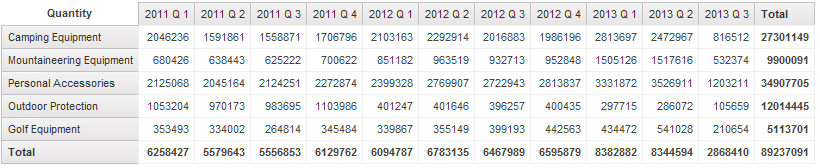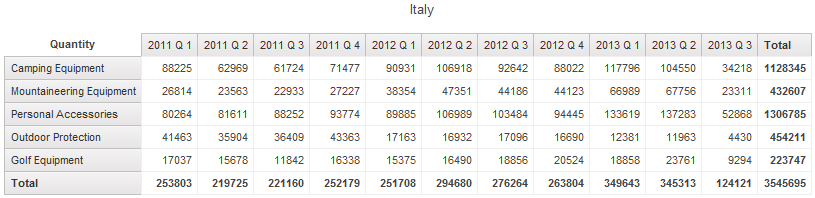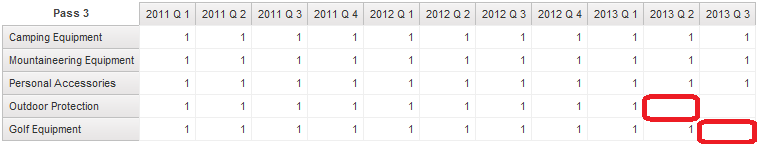# Secondary Cell Suppression on OLAP

I recently had the great pleasure of working with the Department of Health and Human Services in New Hampshire. They have employed a company called Abacus Service Corporation to develop a wonderful dashboarding system in order to assist them in their goals of greater transparency (which I hope to review on here eventually, it’s a combination of Metric Studio and Workspace that is significantly easier for end users). Their team is extremely skilled, and they only needed a few days of my time to get them past a few difficult problems. They’ve agreed to let me publish the solution two of those problems.

One of their goals is to publish reports, directly to the public, on various health related issues. While transparency is to be commended, smaller data sets present possible privacy law violations. If data was sparse enough, people could theoretically back calculate in order to determine who specific people are from the data. In order to prevent people from being able to back calculate, the need to suppress additional data. They were able to accomplish their goal, but at a cost of a runtime of around 15 minutes. For a simple dataset, and one is supposed to be consumable by end users on the fly, any run time of more than a few seconds is unacceptable.

Let’s reproduce a sample using the Sales and Marketing cube. In this example we’ll say that any value below 20,000 and above 0 is sensitive and must be suppressed.In that screenshot we can see the quantity sold for each product line for each quarter. Here we can see that not a single value is below 20,000. However, what happens if we slice by a specific country?Here we see the same data set sliced by Italy. We can instantly see many instances of sensitive data being displayed (remember, anything below 20,000 and above 0 is sensitive). For ease of understanding, I’m going to call rename Quantity as “Check Measure”.
Suppressing those values is a simple matter of:

`if([Check Measure] not between 1 and 20000) then ([Check Measure]) else (null)`

Save that expression in a new data item called Pass 1.Now we can see sensitive cells are suppressed. Unfortunately it is trivial to recalculate those values where only one cell is suppressed in a column, especially considering that we’re showing a totals row. Each column and row must have either >1 or 0 suppressed cells. To make it even easier to understand, I’ll rename Years to Columns and Product Line to Rows.
First thing to do, create a two new items that finds the next minimum value in the columns. We will want to suppress those values in order to secure the suppressed value. The expression is written to use the memberUniqueName in the rows in order to avoid issues with the next value being a tie.

```Columns Min
#/*
This finds the minimum value of Check Measure after the values have been filtered out.
If there are any values suppressed in the first pass, the second pass will filter by values greater than what this returns, effectively suppressing a minimum of 1 more cell than are suppressed.
roleValue(
'_memberUniqueName'
, item(
bottomCount(
[Columns]
, 2
, [Check Measure]
)
, 1
)
)
```

Next, the second pass checks if the current row has one suppressed cell, and if so, it will suppress the cell where the column matches the result from Columns Min

```#/*
2nd Pass
This will count the number of cells in the columns, and deduct the number of cells remaining. If the value is greater than 0, then a cell is being suppressed - suppress any cells which are equal to the minimum value remaining.
*/#

member(if([Pass 1] is null) then ([null]) else (
if(
total([One] within set [Columns]) - total([Pass 1] within set [Columns])=1)
then (
if(roleValue('_memberUniqueName',[Columns]) =[Columns Min]) then ([null]) else ([One])
)
else ([One])
))
```Now the same basic idea for rows.

Rows Min:

```#/*
This finds the minimum value of Check Measure after the values have been filtered out.
If there are any values suppressed in the first pass, the third pass will filter by values greater than what this returns, effectively suppressing a minimum of 1 more cell than are suppressed.
*/#

roleValue(
'_memberUniqueName'
, item(
bottomCount(
[Rows]
, 2
, [Check Measure]
)
, 1
)
)```

and Pass 3:

```#/*
3rd Pass
This will count the number of cells in the rows, and deduct the number of cells remaining. If the value is greater than 0, then a cell is being suppressed - suppress any cells which are equal to the minimum value remaining.
*/#

if([Pass 2]  is null) then ([null]) else (
if(
total([One] within set [Rows]) - total([Pass 1] within set [Rows])=1)

then (
if(roleValue('_memberUniqueName',currentMember(hierarchy([Rows]))) =[Rows Min]) then ([null]) else ([One])
)

else ([One]))```

Again, first it finds all columns that have 1 suppressed cell in the rows then it suppressed the next lowest value:In this case, there are no more instances of unsecured sensitive cells. However, what happens if we had a data set containing only one sensitive cell? It would suppress another cell in the same row and column, but those two cells would now be at risk. If we slice by the United States the third pass returns the following set:One more pass is needed to hide the remaining intersection:

```if([Pass 3] is null) then (null) else (
if ([cc]  = 1) then(
if(roleValue('_memberUniqueName',currentMember(hierarchy([Rows]))) =
roleValue('_memberUniqueName',
order(filter([Rows], ([cr] >0)and ( [Pass 2]  is not null) )  , [Check Measure] ,basc
),1),0)
) ) then (null) else ( [Final Measure])
)
else  ( [Final Measure])
)```

cc is a very simple: total([One] within set [Rows]) – total([Pass 2] within set [Rows])
with cr being: total([One] within set [Columns]) – total([Pass 3] within set [Columns])

This is similar to pass 2. It will count the number of suppressed cells in the column. The big change is instead of looking for and suppressing the smallest value the column, we are now looking for a row that already has at least one suppressed cell. It will find the matching rows, order them ascending by number of suppressed cells, and take the last one in the list. Instead of returning a 1 or null, this will return the final measure, in this case revenue.

And the final results, for the United States:The end result will always be a minimum of 2 rows hidden on each row or column, or none at all. This satisfies the demand for data security on a row level without having to run extremely complex calculations for each possible combination of data in the ETL.

At this point the run time is about 2 seconds (on my laptop), still a long time considering the original query, but far more manageable. It should be mentioned that this is useful for smaller data sets, additional columns and rows will increase the run time significantly. Nested nodes will cause additional complexity, and should probably be avoided.

Sample report can be found here.